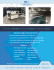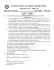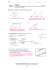# *** Answer Key*** Cumulative Review Worksheet

## Transcription

*** Answer Key*** Cumulative Review Worksheet
```*** Answer Key***
Name: ____________________________________________________ Period:________ Date:____________________
Cumulative Review Worksheet
Rewrite the conditional statement in if-then form.
1. I will go to the game if I get all of my homework done.
If I get all of my homework done, then I will go to the game.
2. A student on the high honor roll has at least a 90 average.
If you have at least a 90 average, then you will be on the high honor roll.
Write the converse, inverse and biconditional of each statement.
6. If you like surfing, then you like to be at the beach. (true)
Converse: If you like to be at the beach, then you like to go surfing. (false)
Inverse: If you don’t like surfing, then you don’t like to be at the beach. (false)
Biconditional: You like to go surfing if and only if you like to go to the beach (false)
7. If angle P is 109 degrees, then angle P is obtuse. (true)
Converse: If angle P is obtuse, then it is 109 degrees. (false)
Inverse: If angle P is not 109 degrees, then it is not obtuse. (false)
Biconditional: Angle P is 109 degrees if and only if angle P is obtuse. (false)
Rewrite the biconditional statement as a conditional statement and its converse. Then decide if the biconditional is true or
false.
8. An angle is acute if and only if it measures less than 90 degrees.
If an angle is acute, then it measures less than 90 degrees.
If an angle measures less than 90 degrees, then it is acute.
9. Three points are collinear if and only if they lie on the same line.
If three points are collinear, then they lie on the same line.
If three points lie on the same line, then they are collinear.
10. M
Mark the figurre to indicate the following
g information
n.
11. O
On a separate piece of papeer, write algeb
braic proofs for
f the followiing equationss.
Proofs will vary, 17)
1 x = 0, 18)) x = -27/2, 20
0) Example below, 21) x = 7/6
Stateement
Reasons
(1/4)x + 2 = 3 – (3
3/4)x
Given
x + 8 = 12 – 3x
Multipliccation properrty of equalityy
4x + 8 = 12
4x = 4
Subtractiion property oof equality
x=1
Divisionn property of eequality
12. F
Find the meassure of each angle.
a
x = 5, y = 11 1) SSet up a systeem: 11xx + 70 = x2+144y – 54 which is equivalentt to X2+ 3y – 124 =0 aand 11xx + 70 + 6y – 22x – 1 = 180 w
which is equivvalent to (11 1 ‐ 9x)/6 = y
Theen substitute the second eequation into the first. 13. B
Brenda has jusst been hired as a plumber’s assistant. Her
H first task iis to open all the water vallves to releasee the pressuree
on thhe lines. She notices that th
he first four valves
v
open by
y turning couunterclockwisse. She conjecctures that alll water valvess
openn by turning co
ounterclockw
wise. Is this inductive or deductive reasooning? Inductive
14. F
Find the missiing angles.
a=200, b= 70, c = 20, d = 70, e = 110
a = 70, c = 225, b = 55
```

### Review12.1-12.6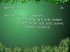### installation of an angle board * * * thermopian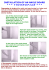### If ABC is obtuse, what are the restrictions on x? ∠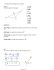### Document 6592969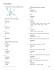### Torque/Cross Product Worksheet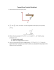### Measuring and Classifying Angles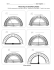### Reteach Sec 1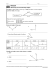### Build an Angle Setting Jig to Sharpening Plane Irons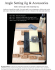### Reflection (from Mulan) Rose is Rose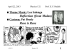### Adjustable Angle Composite Sheeter Options